﻿ Seakeeping Performance Estimation of the Container Ship under Irregular Wave Condition Using Artificial Neural NetworkPublications are Open
Access in this journal
Article Versions
Export Article
• Normal Style
• MLA Style
• APA Style
• Chicago Style
Research Article
Open Access Peer-reviewed

### Seakeeping Performance Estimation of the Container Ship under Irregular Wave Condition Using Artificial Neural Network

Hamid Reza Taghva, Hassan Ghassemi , Hashem Nowruzi
American Journal of Civil Engineering and Architecture. 2018, 6(4), 147-153. DOI: 10.12691/ajcea-6-4-3
Published online: April 18, 2018

### Abstract

In the current study, sea keeping performance of the S-175 container ship is estimated under irregular wave conditions by using numerical calculation and artificial neural networks (ANNs). For this purpose, strip theory is employed to calculate of the response amplitude operator (RAO) and wave resistance. Then, the RAO of heave, pitch, and roll motions and added resistance are used in the considered ANN. In our calculation, the ship dimensions are changed at the same displacement and body form. By comparing the RAO diagrams and according to survey seakeeping criteria, optimum hull is determined for seakeeping performance. In addition, predictive equations based on length of vessel (L), the breadth (B), draft (T) and wave encounter angle (μ), are presented to estimated of seakeeping performance by using ANN.

### 1. Introduction

Estimation of the ships seakeeping performance is one of the most important points in design procedure. In the seakeeping, we calculate the RAO and analyze the body fluctuation in three roll, heave and pitch motions. These fluctuations are related to the body motions including its velocities and accelerations at different body points, and they are affective on the performance of equipment, crew, and thrust systems. In 1949, pioneering study to calculated of body motions was presented by Ursell 1. Sarioz et al 2 conducted some research in the field of practical seakeeping for ship designing. In 1970, Salvesen 3 added the effects of ship motion at a constant speed to the method that provided by Ogilvie and Tuck 4. Simonsen et al 5 analyzed the heave and pitch motions for a KCS ship in regular waves. Bhushan et al 6 used the volume of fluid method to investigate of the effects of free surface for seakeeping calculation. Huang et al 7 confirmed the numerical estimation by seakeeping testing and analyzed the effects of nonlinear slamming and accidental sloshing on general Liquefied Natural Gas (LNG) carrier motions. Otumom and Sener 8 studied the effect of some parameters such as L, B, T,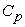and LCB on the seakeeping. By changing these parameters symmetrically, a new body form produced and presented the results. Bagheri et al 9 used the genetic algorithm to optimize body shape for better seakeeping behaviour. In 2015, Guillermo Vásquez et al 10 studied the numerical and experimental results of the vertical motion response of a bulk carrier and a Roll on-Roll of ship. In 2016, Jin et al 11 empirically studied the seakeeping performance of a FLNG liquid gas carrier ship. On the other hands, various investigations have been done using the ANN in the marine field 12, 13, 14, 15. In example, Nowruzi et al 12 predicted the performance of the submerged hydrofoil by using an ANN and CFD. Shora et al. 14 predicted the performance and cavitation volume of submerged propeller under different geometrical and physical characteristics by using CFD and ANNs. Effect of hull form coefficients on the vessel sea-keeping performance and also the parametric on the vessel body lines modeling to optimize seakeeping performance carried out by Khosravi & Ghassemi 15, 16, 17. Bagheri & Ghassemi presented the optimization of Wigley hull form in order to ensure the objective functions of the seakeeping performance 18.

In this paper, preliminary seakeeping performance of the Container ship under irregular waves is studied by investigation of wet frequency of the deck, the frequency of slamming, the acceleration of slamming, the propeller exit from the water and root mean square (RMS) of the vertical acceleration. Then, we used suitable ANNs to predict of the seakeeping behaviour.

### 2. Problem Equations

The ship motion in the wave has six degree of freedom. Among of them, roll, heave and pitch motions are more important in determining whether the seakeeping performance is acceptable or unacceptable. The relationship between body motions and their effect on reducing its desirable performance can be determined by probability. This can only be done by collecting and analyzing large amounts of information obtained from trips of different ships. Thus, more precise definitions of limitations related to different levels of seakeeping performance can be obtained. In this paper, five important criteria of the 12 main criteria of the seakeeping, the exit from the propeller, the frequency of bow slamming, the acceleration of the slamming, the deck wetting and the RMS vertical accelerations for the container S -175 and modeling vessels were studied and compared with the values in Table 2 of Ref. 19. In order to determine the number of decks wetting, slamming and exit of the propeller from the water, Riley relations are used in which the probability of occurrence of each of these criteria is calculated with relations 5-11.The probability of wetting the deck may be calculated as follows: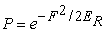(1)

where F is the freeboard, and ER is the area under the relative vertical motion curve at the point under consideration. The mean period is defined as follows: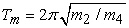(2)

where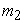is the variance of velocity, and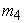is the variance of acceleration. After that, we can use the Eq.(3) to count the occurrence of each of the seakeeping criteria in one hour according to: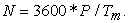(3)

Slamming phenomenon will be occurred when the bottom of the vessel moves from the water under the impacts of the surface of the wave at a minimum VT. This vertical relative velocvity has a vertical relative speed of 3.66 m/s for a 158-meter-long ship at a distance of 15% from the fore perpendicular. Therefore, the probability of bottom moves from water as follows 20: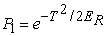(4)

T is a draft at the site of the slamming event. The probability of an impact of the ship bottom to the surface of the wave with a speed exceeding the minimum speed has following form: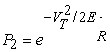(5)

where the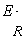is the surface below the curve of the relative velocity spectrum at the point under consideration for slamming. The probability of slamming is as follows: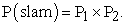(6)

The probability of propeller exiting from water is as follows: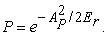(7)

The AP is the distance between the highest points of the propeller blade from the water surface.

### 3. Prediction of the seakeeping Behavior by ANN

ANNs are inspired by the nature of human ANNs, which are composed of united processing units called neurons. These neurons are generally in three layers. As shown in Figure 1, input layer, hidden layers, and output layer are these three layers. On the other hand, one of the most prominent models available for the ANN is the back propagation artificial neural networks. In this manner, the event output (i.e. reference data) will be received by the input layer. These layers are then passed to the input variables. This is done by the transfer function of identity. Then, they enter into hidden layers through the inner connection of the input and hidden layer neurons. The main calculations and processing in the ANN are hidden in the same layers and due to the weights of the neurons. In fact, in the hidden layer neurons, the aggregate outputs of the previous layers are weighed. Then they are weighed by bias, and then this sum passes through the transfer function. In this paper, we used the MATLAB-ANN simulink to predict the ship point data, and use the hyperbolic tangent sigmoid transfer for hidden layer neurons. This function is defined as follows: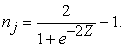(8)
• Figure 1. Multilayer perceptron ANN structure

Here,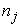is the output of the neuron, and z is also calculated as follows: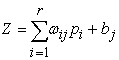(9)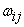is the connecting weights of the i-th neurons of the previous layer to j-th neurons and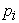is the i-th neuron output. In addition, r and bj are the number of previous neurons in the previous layer and the bias, respectively. In this paper, the linear transfer function (λ) for the output layer is use.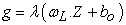(10)

where,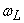is the connecting weights between the last hidden layer and the input layer. In addition, b0 is the last layer bias.

On the other hand, in Feed-Forward Back-Propagation ANNs, the training algorithm is implemented based on forward-facing inputs, post-sharing of common errors, and weight and bias adjustments using error transmission to previous layers. This method of training, known as post-trafficking, was developed by Rumelhart et al. 21. In this manner, the error function is minimized using nonlinear optimization. Also, Marquardt–Levenberg Algorithm has been used to optimize the ANN that the methods for setting network parameters (weights and biases of neurons) and initial random values are considered. On the other hand, there are different uncertainties that lead to errors in the estimation of the ANN. To study the ability ANNs in estimation of considered case, different definitions of accuracy and error are presented by the researchers 22, 23. In this research, two measures of the correlation coefficient (R) and mean square error (MSE) has been used. These two parameters are defined as follows: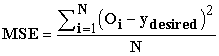(11)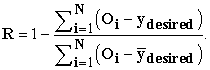(12)

Here, the number of input data, reference data, predicted outcomes and average values of the desired values are specified with N,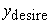,Oi and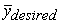, respectively. It should be noted that when the value of R approaches 1, it indicates a good estimation of the ANN. Also, lower MSE values also suggest less error in estimation. In this paper, using 645 numbered simulations, input data into the ANN is provided. These data are then randomly divided into three categories of training data, verification data, and test data. We consider 60% of the data for training as well as 20% for each verification and testing category. The reason is the use of verification and testing data to ensure the ANN. But since the inputs and outputs of the ANN have different values of magnitude, the process of normalizing the data between the ranges of 0.1 to 0.9 was used based on the error study and the following equation is used 24.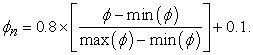(13)

Here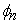is the normalized input value. The ANN is provided for simultaneous estimation of the heave, pitch and roll RAO and added resistance. Also, for finding the desired ANN for each of the characteristics, one layer of ANNs and two-layer ANN scenarios have been considered.

### 4. Results and Discussions

Container ship (S-175) is selected for the present calculations because of many seakeeping data are available 25. Main dimensions and other required data are given in Table 1. The body plan and its three-dimensional are shown in Figure 2. S-175 is operated at Froude number of 0.2 and irregular condition with ITTC wave spectrum. Figure 3 is presented the comparison of the RAO of the heave and pitch, respectively. As can be, the present method results are relatively good agreement with experimental data. In this paper, five criteria (vertical acceleration, slam acceleration, slamming frequency, deck wetness and propeller emergence) for the S-175 at various incidence angles 0, 180,135,160, and vessels modeled at 180 degrees, in the ITTC wave spectrum of two parameters with a height of 8.167 m and a period of waves of 14.47 sec. The results are presented in Table 2.

• Figure 2. Bodyplan (A) and three-Dimensional (B)
• Figure 3. RAO comparison graph of the S-175 vertical displacement motion at μ =180 deg, Fn = 0.2

By comparing the values in Table 2 with the marine seakeeping standards 16, it can be concluded that which of the ships have a better seakeeping performance and meet the criteria for seakeeping behavior. Also, in order to determine the weight and importance of each of the phenomena examined, and because of the search for information sources and scientific articles about the degree of importance and which criteria are more important in improving the performance of seakeeping The vessel was not found. Therefore, to determine the best performance of the seakeeping among the modeling vessels, the following proposed model has been used. Here are five benchmarks to check that the share and value of each criterion are assumed to be equal (0.2), so the contribution of each of the phenomena is 0.2, the sum of which is 1. Using the Eq. (14), we describe the subject.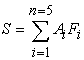(14)

where, F1, F2, F3, F4, F5, respectively, represent 5 seakeeping criteria, RMS vertical accelerations, slamming acceleration, slamming frequency, frequency of the deck wetness and outlet of the propeller from the water (propeller emergence), and values A1, A2, A3, A4, A5 have two values zero or one .zero, meaning that this criterion is not satisfied and one means that this criterion is satisfied. If the value is S = 1 it means that all the criteria are met, and if S = 0 it means that none of the criteria is satisfied and if the value of S = 0-1 satisfies a part of the criteria and part they are not satisfied, respectively, represented by the letters S (satisfied), U (unsatisfied), and P (partial satisfied).

With respect to Table 2 and Table 3, it can be seen intuitively that the sample of the stared ship in Table 4 satisfies all the criteria for seakeeping and have good seakeeping performance. And among all the ships, the ship length of 179.56 has the best performance of seakeeping. The RAO of heave, pitch, roll are met the seakeeping criteria. The RAO of heave, pitch, roll and added resistance related to ships satisfying the criteria for the seakeeping are shown in Figure 4 and Figure 5.

Based on Ref. 12 and with the choice of the desired ANN, one can simultaneously predict all the characteristics of the seakeeping based on different.

• Figure 4. RAO heave and pitch of the S-175 with different lengths at μ =180, and Fn = 0.2, (A) Heave RAO, (B) Pitch RAO
• Figure 5. RAO added resistance of the S-175 with different lengths at μ =180, and Fn = 0.2
• Figure 6. Regression results between the predicted input and output data from the ANN

Based on Figure 6, it can be concluded that the ANN with a negligible error correctly predicts all four characteristics of the seaman. On the other hand, according to the research conducted, it has been determined that there is no predictive equation for predicting all four characteristics of the desired seismic.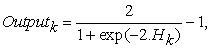(15)

where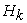is defined as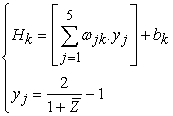(16)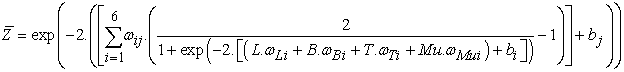(17)

Table 4 is given the coefficients of the proposed defined equations (16) and (17).

### 5. Conclusions

In this paper, seakeeping behavior of the S-175 ship is studied by considering the RAO heave, pitch and added resistance. According to the results, the following conclusions can be drawn:

• The RAO and added resistance results at different operation conditions are presented. it is observed that with a length of 174.04, 174.24 and 179.56 have less resistance value than the S-175 ship.

• Among all the ships, the ship length of 179.56 has the best performance of seakeeping. The RAO of heave, pitch, roll are met the seakeeping criteria.

• Based on the weights and bias extracted using the ANN, an estimated equation for the seakeeping is obtained in terms of the L, B, T and μ.

### References

Published with license by Science and Education Publishing, Copyright © 2018 Hamid Reza Taghva, Hassan Ghassemi and Hashem NowruziThis work is licensed under a Creative Commons Attribution 4.0 International License. To view a copy of this license, visit http://creativecommons.org/licenses/by/4.0/